## Filters

Sort by :
Q
Engineering
90 Views   |

For a regular polygon, let be the radii of the inscribed and the circumscribed circles. A false statement among the following is

• Option 1)

there is a regular polygon with

• Option 2)

there is a regular polygon with

• Option 3)

there is a regular polygon with

• Option 4)

there is a regular polygon with

As we learnt in Relation between Systems of Measurement of Angles - - wherein D  Angle in Degrees G  Angle in Grades C  Angle in Radians    Let O be centre of Polygon, AB be any edge. In radius r = OC Then R = OA = OB,    .                       AB = a Then In    In radius   Similarly, In          {divides (1) by (2)} Option 1) there is a regular polygon with  Incorrect Option...
Engineering
118 Views   |

Let   and let , where   Then

• Option 1)

• Option 2)

• Option 3)

• Option 4)

As we leant in Double Angle Formula - - wherein These are formulae for double angles.     Adding (1) and (2)    Option 1) This option is incorrect. Option 2) This option is correct. Option 3) This option is incorrect. Option 4) This option is incorrect
Engineering
114 Views   |

Let   Then the vector satisfying

• Option 1)

• Option 2)

• Option 3)

• Option 4)

As we learnt in  Unit vector perpendicular to the plane of vector a and b - - wherein Here  and  are two vectors.     Scalar Product of two vectors (dot product) - - wherein  is the angle between the vectors     So  Option 1) This option is correct. Option 2) This option is incorrect. Option 3) This option is incorrect. Option 4) This option is incorrect.
Engineering
113 Views   |

If the vectors and are mutually orthogonal, then

• Option 1)

( -3, 2 )

• Option 2)

( 2, -3 )

• Option 3)

( -2, 3 )

• Option 4)

( 3, -2 )

As we learnt in  Scalar Product of two vectors - - wherein   is the angle between the vectors     For mutually orthogonal, On solving Option 1) ( -3, 2 ) This option is correct. Option 2) ( 2, -3 ) This option is incorrect. Option 3) ( -2, 3 ) This option is incorrect. Option 4) ( 3, -2 ) This option is incorrect.
Engineering
142 Views   |

Directions : Question are Assertion- Reason type questions. Each of these questions contains two statements. Statement-1 (Assertion) and Statement-2 (Reason). Each of these questions also has four alternative choices,only one of which is the correct answer. You have to select the correct choice:

Statement-1 : The point (3, 1, 6) is the mirror image of the point (1, 3, 4) in the plane

Statement-2 : The plane bisects the line segment joining (3, 1, 6) and (1, 3, 4).

• Option 1)

Statement -1 is true, statement -2 is true ; statement -2 is a correct explanation of statement-1.

• Option 2)

Statement -1 is true, statement -2 is true ; statement -2 is not a correct explanation of statement-1.

• Option 3)

Statement -1 is true, statement -2 is false.

• Option 4)

Statement -1 is false, statement -2 is true.

As we learnt in  Image of a point - Let be the image of point in the plane will be given by the formula  -   Statement 1 is true as well. But it is not necessary that if a plane bisects line joining A and B, that B is the image of A.   Option 1) Statement -1 is true, statement -2 is true ; statement -2 is a correct explanation of statement-1. Incorrect option Option 2) Statement -1 is...
Engineering
149 Views   |

A line  AB in three-dimensional space makes angles 450 and 120 with the positive -axis and the positive -axis respectively. If AB makes an acute angle with the positive -axis , than equals

• Option 1)

300

• Option 2)

450

• Option 3)

600

• Option 4)

750

As we learnt in Direction Cosines - i)    ii)    If OP =r then the co-ordinates of P will be (lr,mr,nr) iii)    Direction cosines of X-axis are (1,0,0) iv)    Direction cosines of Y-axis are (0,1,0) v)    Direction cosines of Z-axis are (0,0,1) -    Direction angles, Given  We know that,    Option 1) 300 Incorrect answer Option 2) 450 Incorrect answer Option 3) 600 Correct answer Option...
Engineering
133 Views   |

Directions : Question are Assertion- Reason type questions. Each of these questions contains two statements. Statement-1 (Assertion) and Statement-2 (Reason). Each of these questions also has four alternative choices,only one of which is the correct answer. You have to select the correct choice:

Question : Let be a 2 × 2 matrix with non-zero entries and let    where is 2 × 2 identity matrix.   Define          sum of diagonal elements of  and = determinant of matrix .

Statement-1 : 0

Statement-2 : = 1

• Option 1)

Statement -1 is true, statement -2 is true ; statement -2 is a correct explanation of statement-1.

• Option 2)

Statement -1 is true, statement -2 is true ; statement -2 is not a correct explanation of statement-1.

• Option 3)

Statement -1 is true, statement -2 is false.

• Option 4)

Statement -1 is false, statement -2 is true.

As we learnt in

Multiplication of matrices -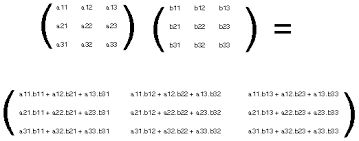-

Option 1)

Statement -1 is true, statement -2 is true ; statement -2 is a correct explanation of statement-1.

Incorrect Option

Option 2)

Statement -1 is true, statement -2 is true ; statement -2 is not a correct explanation of statement-1.

Incorrect Option

Option 3)

Statement -1 is true, statement -2 is false.

Correct Option

Option 4)

Statement -1 is false, statement -2 is true.

Incorrect Option

View More
Engineering
237 Views   |

The number of 3 x 3 non-singular matrices, with four entries as 1 and all other entries as 0, is

• Option 1)

less than 4

• Option 2)

5

• Option 3)

6

• Option 4)

at least 7

As we learnt in

Defination of Matrix -

A rectangular array of symbols (which could be real or complex numbers) along rows and columns is a matrix.

-

Use combination method and 4 entries for 1 and others for zero. Then atleast 7 number of non-singular matrices

Option 1)

less than 4

Incorrect Option

Option 2)

5

Incorrect Option

Option 3)

6

Incorrect Option

Option 4)

at least 7

Correct Option

View More
Engineering
103 Views   |

Consider the system of linear equations

The system has

• Option 1)

infinite number of solutions

• Option 2)

exactly 3 solutions

• Option 3)

a unique solution

• Option 4)

no solution

As we learnt in

Cramer's rule for solving system of linear equations -

When  and atleast one of     is non-zero , system of equations has no solution

- wherein

and

Option 1)

infinite number of solutions

Incorrect Option

Option 2)

exactly 3 solutions

Incorrect Option

Option 3)

a unique solution

Incorrect Option

Option 4)

no solution

Correct Option

View More
Engineering
147 Views   |

Let    be defined by

If has a local minimum at , then a possible value of is

• Option 1)

1

• Option 2)

0

• Option 3)

–1/2

• Option 4)

–1

As we learnt in Differentiability - Let  f(x) be a real valued function defined on an open interval (a, b) and   (a, b).Then  the function  f(x) is said to be differentiable at      if -     Since f (x) is differential so it must be continuous. Option 1) 1 This option is incorrect. Option 2) 0 This option is incorrect. Option 3) –1/2 This option is incorrect. Option 4) –1 This option is correct.
Engineering
103 Views   |

The equation of the tangent to the curve that is parallel to the -axis, is

• Option 1)

• Option 2)

• Option 3)

• Option 4)

As we learnt in Rate Measurement - Rate of any of variable with respect to time is rate of measurement. Means according to small change in time how much other factors change is rate measurement: - wherein Where dR / dt  means Rate of change of radius.       Option 1) This option is incorrect. Option 2) This option is incorrect. Option 3) This option is incorrect. Option 4) This...
Engineering
117 Views   |

Let be a positive increasing function with

• Option 1)

1

• Option 2)

2/3

• Option 3)

3/2

• Option 4)

3

As we learnt in Evaluation of limits : (algebraic limits) : (Method of direct substitution) - - wherein Means at  x = a  f(x) defined.        Since f(x) is increasing function so that      Option 1) 1 This option is correct. Option 2) 2/3 This option is incorrect. Option 3) 3/2 This option is incorrect. Option 4) 3 This option is incorrect.
Engineering
101 Views   |

Let S be a non-empty subset of R. Consider the following statement:

P : There is a rational number such that

Which of the following statements is the negation of the statement P ?

• Option 1)

There is a rational number such that .

• Option 2)

There is no rational number such that .

• Option 3)

Every rational number satisfies  .

• Option 4)

and is not rational.

As we learnt in  Negation of a Statement - Symbol of negation is  - wherein We write it up as p     There is rational numbers    such that  negation is every rational number   satisfies Since negation of is Option 1) There is a rational number such that .    Incorrect option Option 2) There is no rational number such that . Incorrect option Option 3) Every rational number satisfies ...
Engineering
121 Views   |

The line L given by   passes through the point (13, 32). The line K is parallel to L and has the equation Then the distance between L and K is

• Option 1)

• Option 2)

• Option 3)

• Option 4)

As we learnt in  Condition for parallel lines - - wherein Here are the slope of two lines    and Distance between two parallel lines -   - wherein is the distance between   and     Put (13, 32) So, m = 4 Also,  Equation is -4x + y = 3 Distance  Option 1) This option is incorrect. Option 2) This option is incorrect. Option 3) This option is incorrect. Option 4) This option is correct.
Engineering
98 Views   |

If two tangents drawn from a point P to the parabola are at right angles, then the locus of P is

• Option 1)

• Option 2)

• Option 3)

• Option 4)

As we learnt in Equation of tangent -   - wherein Tangent at       and Standard equation of parabola - - wherein   Locus of perpendicular tangents is the directrix. so, x = -a i.e. x = -1  Option 1) This option is incorrect. Option 2) This option is incorrect. Option 3) This option is correct. Option 4) This option is incorrect.
Engineering
98 Views   |

The circle   intersects the line at two distinct points if

• Option 1)

• Option 2)

• Option 3)

• Option 4)

As we learnt in  Condition of tangency -   - wherein If    is a tangent to the circle     On solving, we get Option 1) THis option is incorrect Option 2) THis option is correct Option 3) THis option is incorrect Option 4) THis option is incorrect
Engineering
132 Views   |

The correct sequence which shows decreasing order of the ionic radii of the element is

• Option 1)

• Option 2)

• Option 3)

• Option 4)

As we learnt in   Size of isoelectronic species - Smaller the value of  z/e, larger the size of that species.Smaller z means effective nuclear charge is small hence size is large. -     For isoelectronic species, as effective nuclear charge increases, ionic radii decreases. Nuclear charge is maximum of the specie with maximum protons . Order of nuclear charge:                    Protons:    ...
Engineering
283 Views   |

The main product of the following reaction is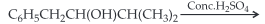• Option 1)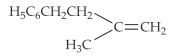• Option 2)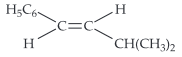• Option 3)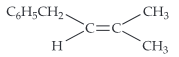• Option 4)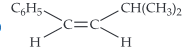As we learnt in  The major product formed is in accordance wtihSaytzeff rule i.e. more substituted alkene is the major product.  Option 1) This option is incorrect Option 2) This option is correct Option 3) This option is incorrect Option 4) This option is incorrect
Engineering
137 Views   |

From amongst the following alcohols the one that would react fastest with conc .HCl and anhydrous ZnCl2, is

• Option 1)

1-Butanol

• Option 2)

2-Butanol

• Option 3)

2-Methylpropan-2-ol

• Option 4)

2-Methylpropanol

The reagent, conc.HCl and anhydrous ZnCl2 is Lucas reagent, which is used to distinguish between 1°, 2° and 3° alcohols.

3° alcohol + Lucas reagent → Immediate turbidity.
2° alcohol + Lucas reagent → Turbidity after 5 mins.

1° alcohol + Lucas reagent → No reaction.
Thus, the required alcohol is 2-methylpropan-2- ol, i.e.View More
Engineering
153 Views   |

The polymer containing strong intermolecular forces e.g., hydrogen bonding is

• Option 1)

natural rubber

• Option 2)

teflon

• Option 3)

nylon-6,6

• Option 4)

polystyren

As we learnt in Fibres - The intermolecular forces between the chains are H - bonds.They are crystalline in nature.  - wherein Eg:-    Nylon 66,  Terylene, Silk.    In Nylon- 6,6, the intermolecular forces between the chains are hydrogen bondings.   Option 1) natural rubber This option is incorrect. Option 2) teflon This option is incorrect. Option 3) nylon-6,6 This option is correct. Option...
Exams
Articles
Questions